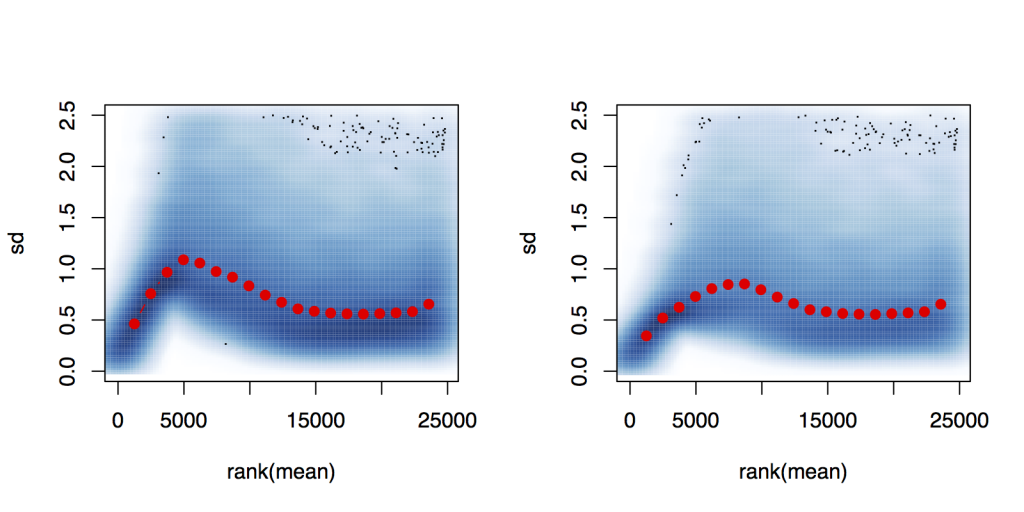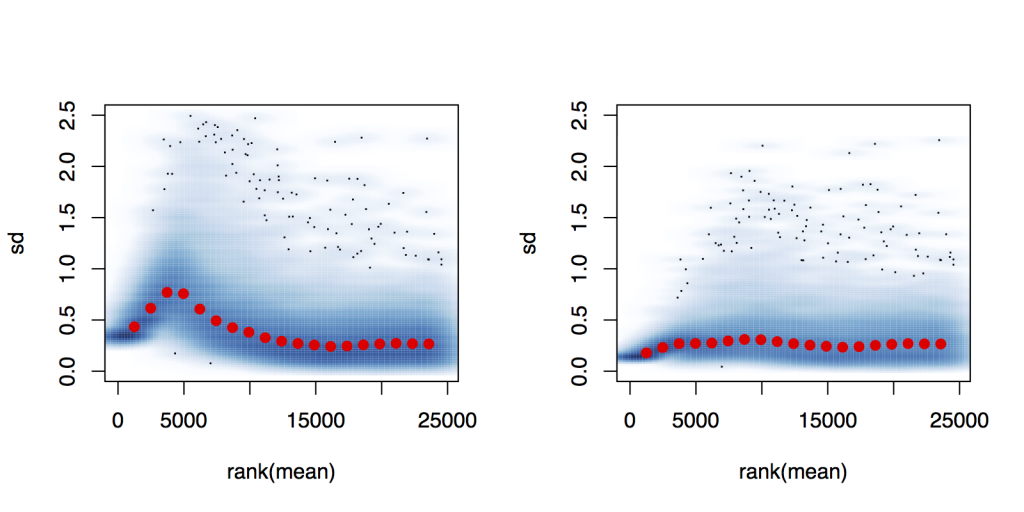View Single Post05-07-2013, 01:02 AM #1 john_nl Member   Location: UK Join Date: Feb 2012 Posts: 13DESeq Variance Stabilizing Transformation Hello, I am looking for some feedback regarding the use of the variance-stabilization (VST) methods found in the DESeq2 package. Hopefully one of the authors will respond and the comments will be of help to others. For me, the purpose for applying this transformation is to be able to generate moderated fold changes for clustering of genes (not samples as in the vignette). My data consists of a time series, where for each time point there is a "treated" sample and a "control" sample. Each sample (timepoint) consists of 4 biological replicates. I performed the VST on the entire set of data and plot the per-gene standard deviation against the rank of the mean*, for the shifted logarithm log2 (n + 1) (left) and the variance stabilizing transformation (right), it does not appear to have a pronounced effect.However, if i set up a count dataset that consists of the samples corresponding to one timepoint only (first timepoint in the example below), and perform the VST and plot the standard deviation against rank of the mean, the transformed values have a much better stabilized standard deviation.So my questions are: Is there anyway to obtain better variance stabilized data when considering the entire timeseries? Should I just perform the VST on a per timepoint basis; after all I will only be computing fold changes between treatment and control samples at the same timepoint. *The procedure was performed as per the DESeq2 manual: dds <- estimateSizeFactors(dds) dds <- estimateDispersions(dds) vsd <- varianceStabilizingTransformation(dds) par(mfrow=c(1,2)) plot(rank(rowMeans(counts(dds))), genefilter::rowVars(log2(counts(dds)+1)), main="log2(x+1) transform") plot(rank(rowMeans(assay(vsd))), genefilter::rowVars(assay(vsd)), main="VST")